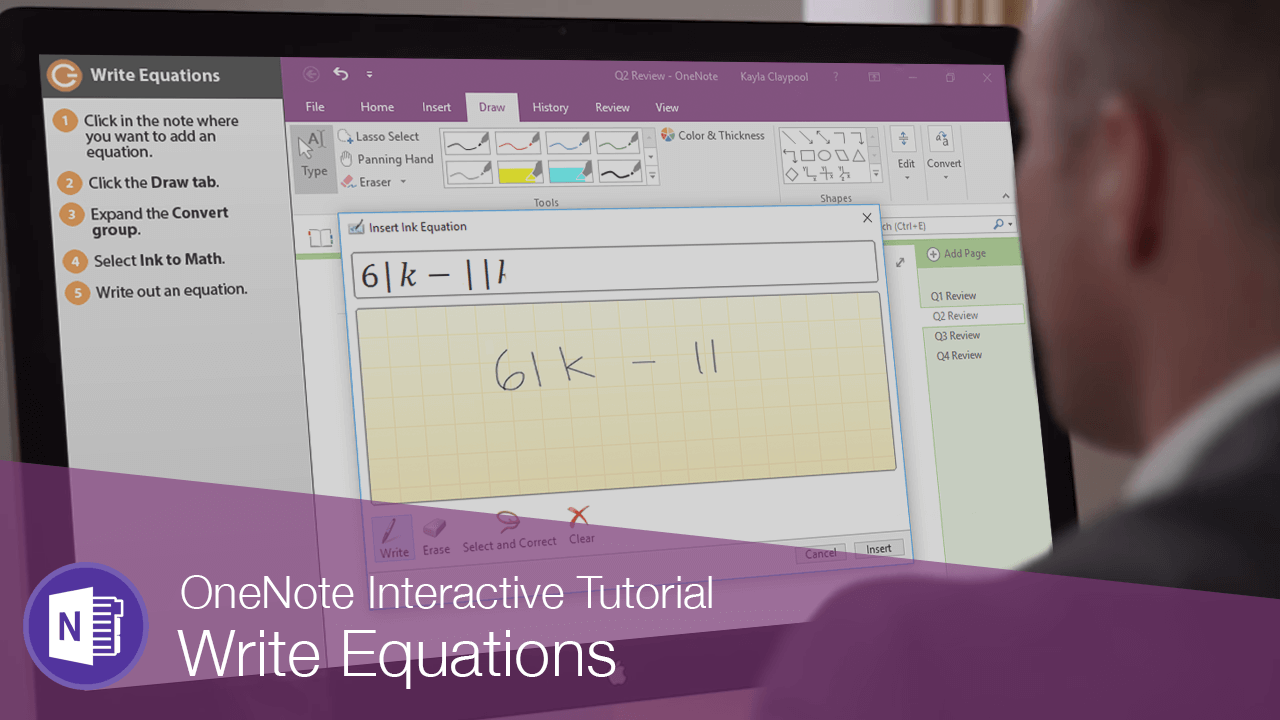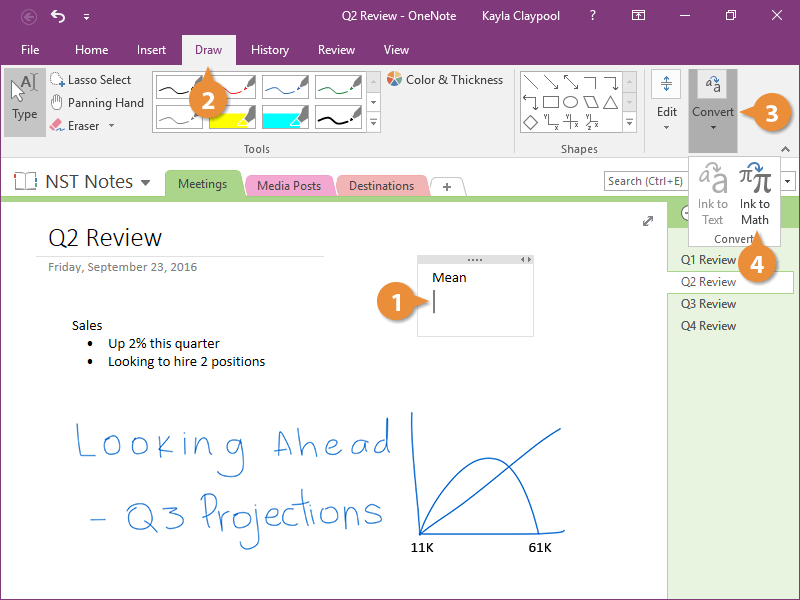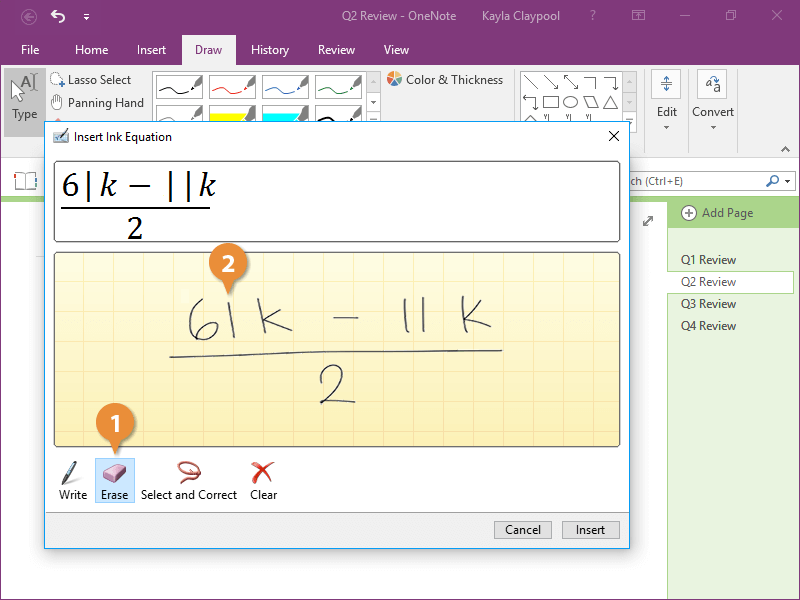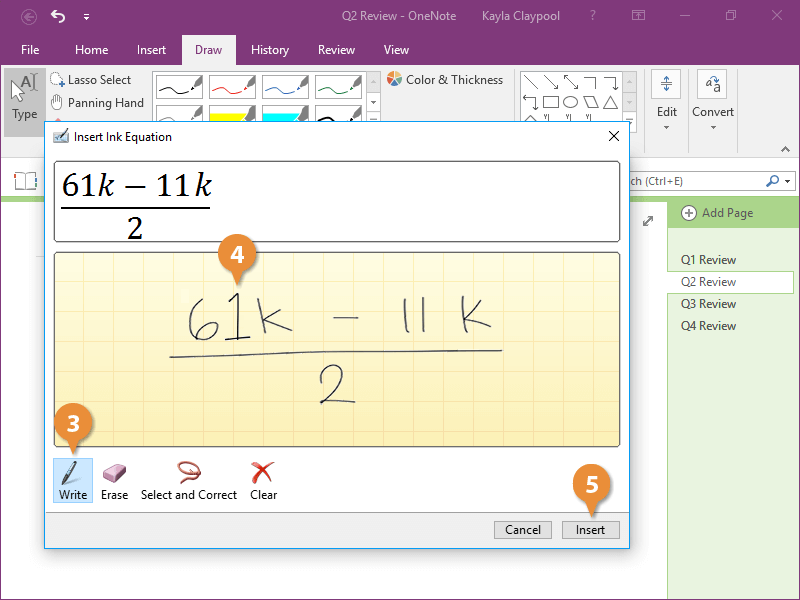Write Equations | CustomGuide# Write Equations

How to Write Equations in OneNoteSometimes in your notes you'll need to add an equation, which can be real tricky if you try to type it. Instead, enlist the help of the Ink to Math feature.

### Write Equations

1. Click in the note where you want to add an equation.
2. Click the Draw tab.
3. Expand the Convert group.
4. Select Ink to Math.The cursor automatically switches to writing mode and you can start writing out the equation

5. Write out an equation.

As you write, OneNote converts your numbers and symbols to type on the fly.

### Fix Errors

You'll notice as you write that the type conversion isn't always correct.

1. Click the Erase button.
2. Click any errors.3. Click the Write button.
4. Re-write the letter or number.
5. Click Insert.The formula is added to the page and it looks a lot better than if you had tried to type it.